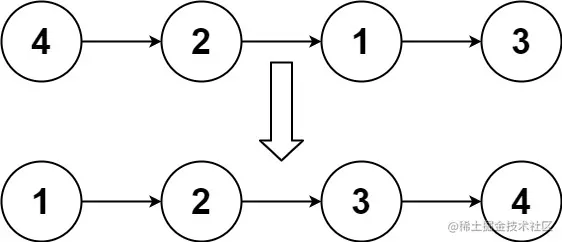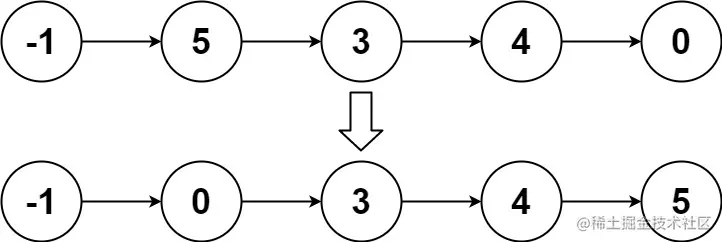# leetcode 148. Sort List（python）

2022-02-01 14:40:57

「 This is my participation 11 The fourth of the yuegengwen challenge 29 God , Check out the activity details ：2021 One last more challenge

### describe

Given the head of a linked list, return the list after sorting it in ascending order.Can you sort the linked list in O(n logn) time and O(1) memory (i.e. constant space)?

Example 1:``````Input: head = [4,2,1,3]
Output: [1,2,3,4]
Copy code ``````

Example 2:``````Input: head = [-1,5,3,4,0]
Output: [-1,0,3,4,5]
Copy code ``````

Example 3:

``````Input: head = []
Output: []
Copy code ``````

Note:

``````The number of nodes in the list is in the range [0, 5 * 10^4].
-10^5 <= Node.val <= 10^5
Copy code ``````

### analysis

According to the meaning , Is to give the head node of a linked list head , Ask us to sort them in ascending order . The title also requires us to use O(n logn) Time complexity and O(1) Of memory . I tried to insert with the simplest violence , Spatial complexity or O(1), however O(n^2) Time complexity , It's over time .

``````class ListNode(object):
def __init__(self, val=0, next=None):
self.val = val
self.next = next
class Solution(object):
"""
:rtype: ListNode
"""
L = ListNode(val=-1000000)
while cur.next:
pre = L
if cur.next.val >= cur.val:
cur = cur.next
continue
while pre.next.val < cur.next.val:
pre = pre.next
tmp  = cur.next
cur.next = tmp.next
tmp.next = pre.next
pre.next = tmp
return L.next

Copy code ``````

### Running results

``````Time Limit Exceeded
Copy code ``````

### analysis

Because the built-in function is used here sorted Sort values , Re create a new linked list , So the time complexity is O(n logn) But I passed the shame . But the spatial complexity is O(n).

``````class ListNode(object):
def __init__(self, val=0, next=None):
self.val = val
self.next = next
class Solution(object):
"""
:rtype: ListNode
"""
node = ListNode(val = 0)
result = node
nlist = []
nlist = sorted(nlist)
for n in nlist:
node.next = ListNode(val = n)
node = node.next
return result.next

Copy code ``````

### Running results

``````Runtime: 284 ms, faster than 84.91% of Python online submissions for Sort List.
Memory Usage: 63.3 MB, less than 12.52% of Python online submissions for Sort List.
Copy code ``````

### analysis

You can also use merge sort , As long as the time complexity is O(n logn) Other sorting algorithms can .

``````class ListNode(object):
def __init__(self, val=0, next=None):
self.val = val
self.next = next
class Solution(object):
"""
:rtype: ListNode
"""
another = mid.next
mid.next = None

while fast.next and fast.next.next:
fast = fast.next.next
slow = slow.next
return slow

def merge(self, A, B):
dummy = cur = ListNode(0)
while A and B:
if A.val > B.val:
cur.next = B
B = B.next
else:
cur.next = A
A = A.next
cur = cur.next
if A: cur.next = A
if B: cur.next = B
return dummy.next

Copy code ``````

### Running results

``````Runtime: 544 ms, faster than 44.25% of Python online submissions for Sort List.
Memory Usage: 45.6 MB, less than 86.45% of Python online submissions for Sort List.
Copy code ``````